Home > A2C > Chapter 7 > Lesson 7.1.4 > Problem7-54

7-54.
1. If two quantities are equal, are their logarithms also equal? Consider the questions below. Homework Help ✎

1. Is it true that 42 is equal to 24? Is this a special case, or is ab equal to ba for any values of a and b?

2. Is log 42 equal to log 24? How can you be sure?

3. Are the equations x = 5 and log x = log 5 equivalent? Justify your answer.

4. Is the equation log 7 = log x2 equivalent to the equation 7 = x2? How can you be sure?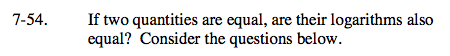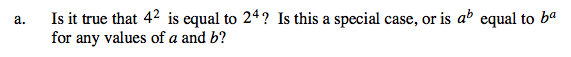Try a few other combinations of ab and ba.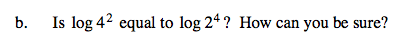Simplify the exponential expressions first.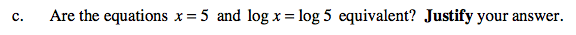What solutions satisfy each equation?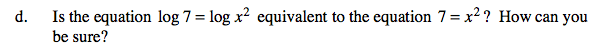See part (c).